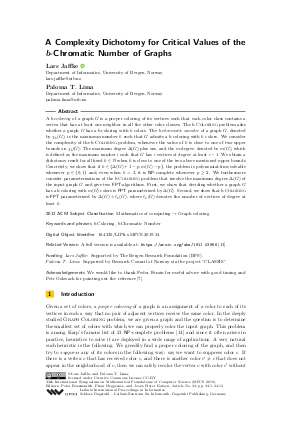Document# A Complexity Dichotomy for Critical Values of the b-Chromatic Number of Graphs

### Authors Lars Jaffke, Paloma T. Lima## File

LIPIcs.MFCS.2019.34.pdf
• Filesize: 0.63 MB
• 13 pages

## Acknowledgements

We would like to thank Fedor Fomin for useful advice with good timing and Petr Golovach for pointing out the reference [Dabrowski et al., 2015].

## Cite As

Lars Jaffke and Paloma T. Lima. A Complexity Dichotomy for Critical Values of the b-Chromatic Number of Graphs. In 44th International Symposium on Mathematical Foundations of Computer Science (MFCS 2019). Leibniz International Proceedings in Informatics (LIPIcs), Volume 138, pp. 34:1-34:13, Schloss Dagstuhl - Leibniz-Zentrum für Informatik (2019)
https://doi.org/10.4230/LIPIcs.MFCS.2019.34

## Abstract

A b-coloring of a graph G is a proper coloring of its vertices such that each color class contains a vertex that has at least one neighbor in all the other color classes. The b-Coloring problem asks whether a graph G has a b-coloring with k colors. The b-chromatic number of a graph G, denoted by chi_b(G), is the maximum number k such that G admits a b-coloring with k colors. We consider the complexity of the b-Coloring problem, whenever the value of k is close to one of two upper bounds on chi_b(G): The maximum degree Delta(G) plus one, and the m-degree, denoted by m(G), which is defined as the maximum number i such that G has i vertices of degree at least i-1. We obtain a dichotomy result for all fixed k in N when k is close to one of the two above mentioned upper bounds. Concretely, we show that if k in {Delta(G) + 1 - p, m(G) - p}, the problem is polynomial-time solvable whenever p in {0, 1} and, even when k = 3, it is NP-complete whenever p >= 2. We furthermore consider parameterizations of the b-Coloring problem that involve the maximum degree Delta(G) of the input graph G and give two FPT-algorithms. First, we show that deciding whether a graph G has a b-coloring with m(G) colors is FPT parameterized by Delta(G). Second, we show that b-Coloring{} is FPT parameterized by Delta(G) + l_k(G), where l_k(G) denotes the number of vertices of degree at least k.

## Subject Classification

##### ACM Subject Classification
• Mathematics of computing → Graph coloring
##### Keywords
• b-Coloring
• b-Chromatic Number

## Metrics

• Access Statistics
• Total Accesses (updated on a weekly basis)
0